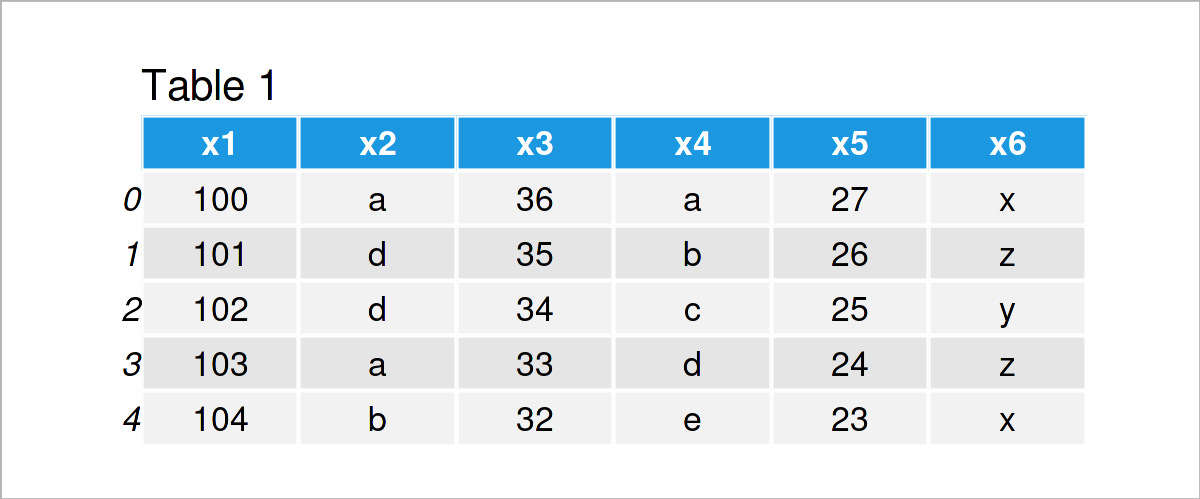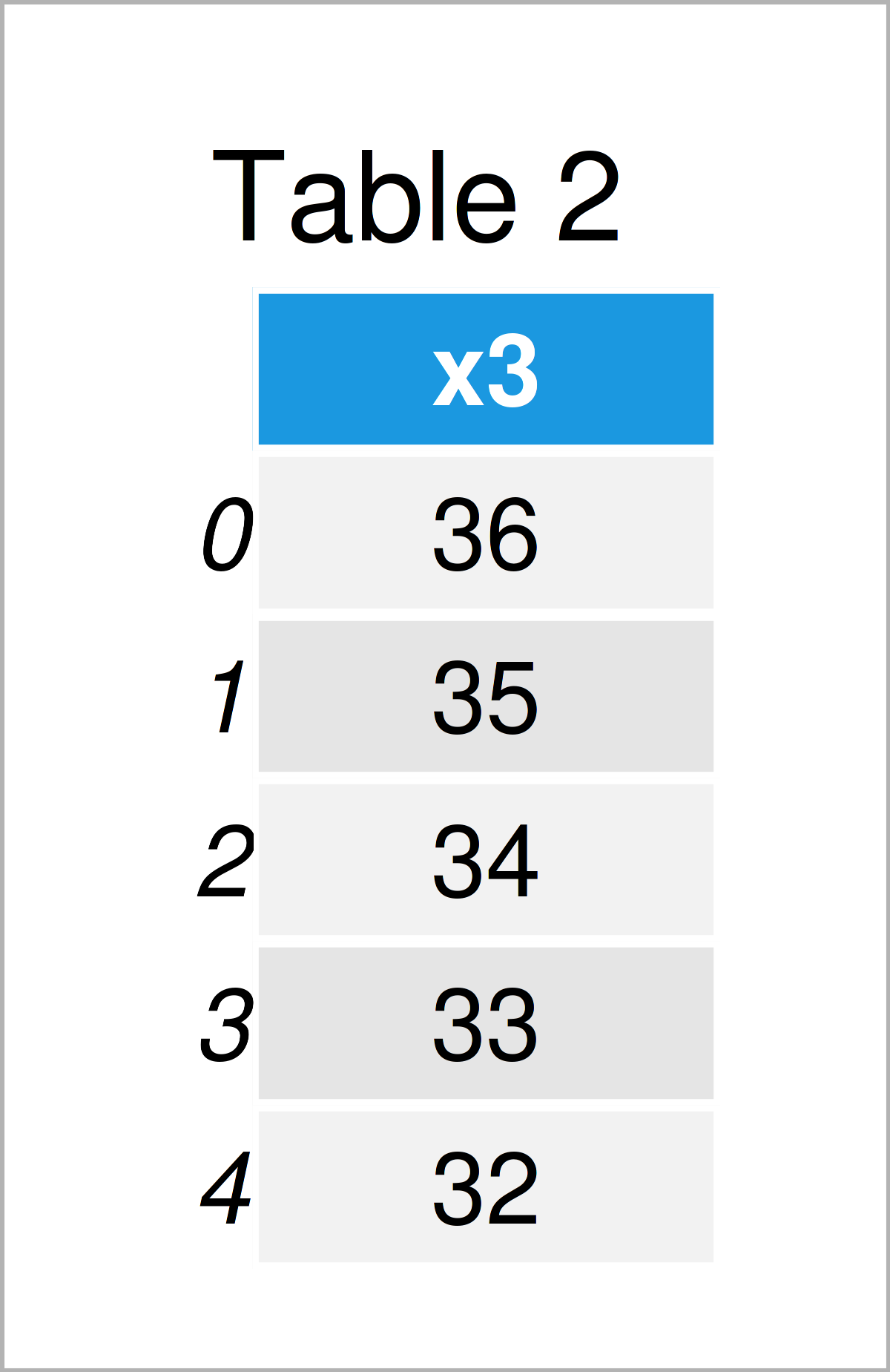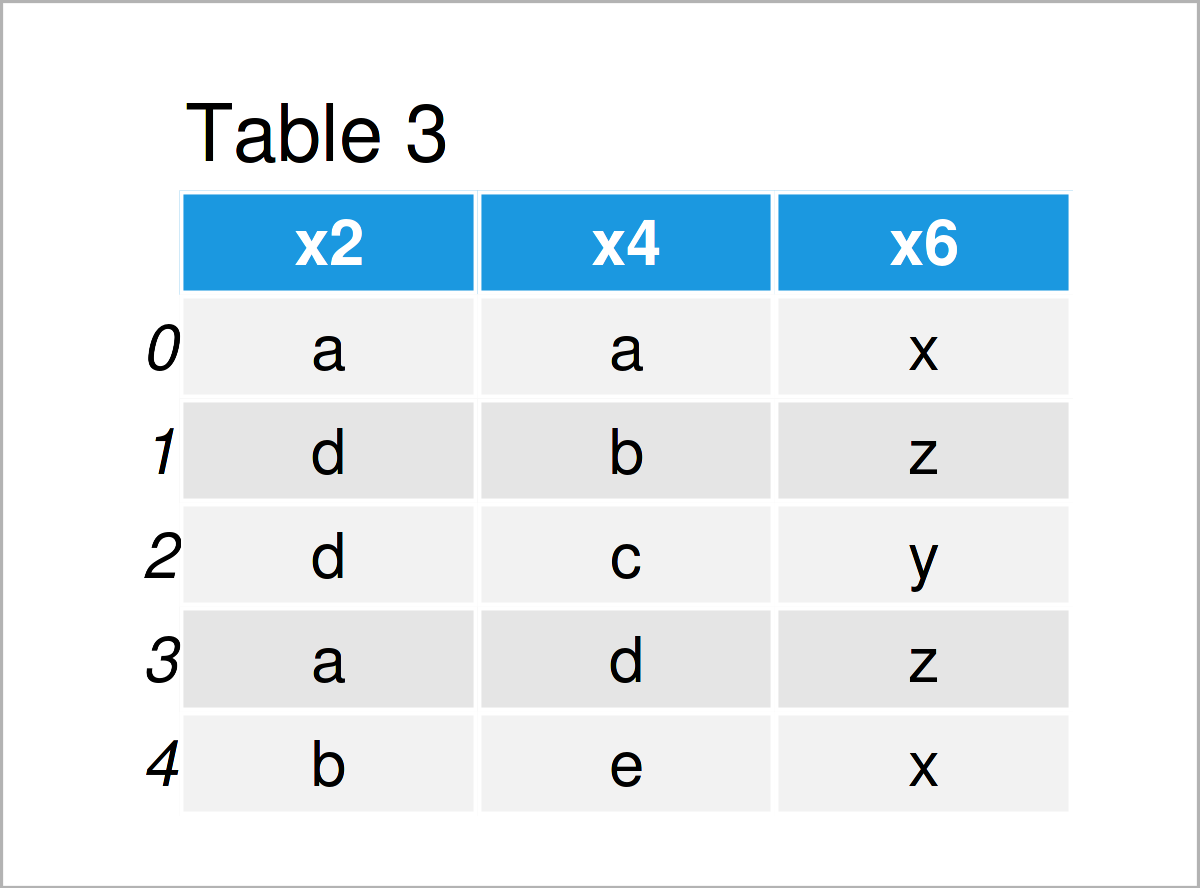# Select Columns of pandas DataFrame by Index in Python (2 Examples)

In this article you’ll learn how to extract particular pandas DataFrame columns by their index position in Python programming.

The page will contain the following information:

Let’s start right away!

## Example Data & Add-On Libraries

In order to use the functions and commands of the pandas library, we first have to import pandas.

`import pandas as pd                           # Import pandas library to Python`

We also have to define some data that we can use in the following examples:

```data = pd.DataFrame({'x1':range(100, 105),    # Create pandas DataFrame
'x2':['a', 'd', 'd', 'a', 'b'],
'x3':range(36, 31, - 1),
'x4':['a', 'b', 'c', 'd', 'e'],
'x5':range(27, 22, - 1),
'x6':['x', 'z', 'y', 'z', 'x']})
print(data)                                   # Print pandas DataFrame```Table 1 shows the structure of our example pandas DataFrame: It is constructed of five lines and six columns.

## Example 1: Extract One pandas DataFrame Column by Index

In this example, I’ll illustrate how to select one particular variable from a pandas DataFrame by its index position in Python.

To accomplish this, we can use the iloc indexer as shown in the following Python syntax:

```data_new1 = data.iloc[:, ]                 # Get one variable
print(data_new1)                              # Print DataFrame with one variable```As shown in Table 2, we have created a new pandas DataFrame that consists of only a single specific variable (i.e. x3).

## Example 2: Extract Multiple pandas DataFrame Columns by Index

In Example 2, I’ll illustrate how to get multiple variables from a pandas DataFrame by indices.

As a first step, we have to define a list of integers that correspond to the index locations of the columns we want to return:

```col_select = [1, 3, 5]                        # Specify indices of columns to select
print(col_select)                             # Print list of indices
# [1, 3, 5]```

In the next step, we can use the iloc indexer and our list of indices to extract multiple variables from our pandas DataFrame:

```data_new2 = data.iloc[:, col_select]          # Get multiple variables
print(data_new2)                              # Print DataFrame with multiple variables```As shown in Table 3, the previous Python programming code has created another pandas DataFrame containing a subset of three variables from our input data set.

## Video & Further Resources

Would you like to know more about the extraction of one or multiple pandas DataFrame variables by index? Then I recommend watching the following video that I have published on my YouTube channel. I illustrate the Python programming codes of this article in the video.

Please accept YouTube cookies to play this video. By accepting you will be accessing content from YouTube, a service provided by an external third party.If you accept this notice, your choice will be saved and the page will refresh.

Furthermore, you might want to read the related Python programming articles on my website: